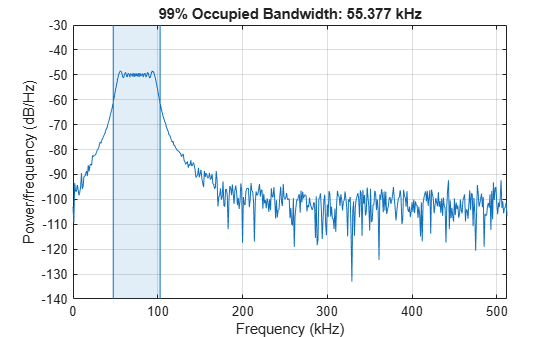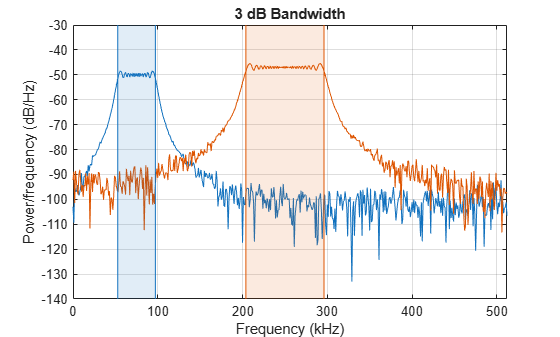# Measure Mean Frequency, Power, Bandwidth

Generate 1024 samples of a chirp sampled at 1024 kHz. The chirp has an initial frequency of 50 kHz and reaches 100 kHz at the end of the sampling. Add white Gaussian noise such that the signal-to-noise ratio is 40 dB.

```nSamp = 1024; Fs = 1024e3; SNR = 40; t = (0:nSamp-1)'/Fs; x = chirp(t,50e3,nSamp/Fs,100e3); x = x+randn(size(x))*std(x)/db2mag(SNR);```

Estimate the 99% occupied bandwidth of the signal and annotate it on a plot of the power spectral density (PSD).

`obw(x,Fs);`Compute the power in the band and verify that it is 99% of the total.

```[bw,flo,fhi,powr] = obw(x,Fs); pcent = powr/bandpower(x)*100```
```pcent = 99 ```

Generate another chirp. Specify an initial frequency of 200 kHz, a final frequency of 300 kHz, and an amplitude that is twice that of the first signal. Add white Gaussian noise.

```x2 = 2*chirp(t,200e3,nSamp/Fs,300e3); x2 = x2+randn(size(x2))*std(x2)/db2mag(SNR);```

Add the two chirps to form a new signal. Plot the PSD of the signal and annotate its median frequency.

`medfreq([x+x2],Fs);`Plot the PSD and annotate the mean frequency.

`meanfreq([x+x2],Fs);`Now consider each chirp to represent a separate channel. Estimate the mean frequency of each channel. Annotate the mean frequencies on a plot of the PSDs.

`meanfreq([x x2],Fs)````ans = 1×2 105 × 0.7503 2.4999 ```

Estimate the half-power bandwidth of each channel. Annotate the 3-dB bandwidths on a plot of the PSDs.

`powerbw([x x2],Fs)````ans = 1×2 104 × 4.4386 9.2208 ```